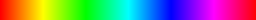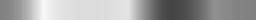# HSL

Even though most human eyes detect colors similarly to how the RGB model works, we normally do not think or talk about colors as a mixture of these three components. We might, however, talk of colors as being more or less saturated than others, having different hues or tones, or as being brighter than others. For this reason, many software applications (open-source examples are Blender, GIMP, Inkscape, and Krita) include color pickers which try to cater to our perception of colors in the terms mentioned above [JG78, p. 21].

One such perceptual color model is HSL (also called HLS [BB09b, p. 207]), which is an acronym for hue, saturation, and lightness. Originally, it was introduced by Joblove and Greenberg as "hue/chroma/intensity" [JG78, pp. 22f.]. They describe the color space as a biconal solid similar to the figure below in which the vertical axis represents all shades of gray between 0 (black) and 1 (white). All fully saturated colors then lie on the outer circle of the common basis of both cones at $$L=0.5$$, which allows for the hue to be defined as an angle. The third parameter, saturation, corresponds to the radius of the circle around the vertical axis at the position of the current lightness.The HSL color space.
Click and drag to rotate, scroll to zoom. On a touch screen, drag to rotate around the y-axis and use two fingers to zoom or to rotate around the x-axis.
Recommended exercises for this section:
• Color matching:
• Color selection:

## Conversion

Given an RGB color $$(r,g,b)_\text{RGB}$$, the lightness $$l$$ and saturation $$s_\text{HSL}$$ of an HSL color $$(h,s_\text{HSL},l)_\text{HSL}$$ are defined as follows [BB09b, p. 212f.]:

\begin{equation} l = \frac{\max\lbrace r,g,b \rbrace + \min\lbrace r,g,b \rbrace}{2} \label{eq:hsllightness} \end{equation}
\begin{equation} s_\text{HSL} = \begin{cases} 0 & \text{if } l = 0 \\ 0.5 \cdot \frac{\max\lbrace r,g,b \rbrace - \min\lbrace r,g,b \rbrace}{l} & \text{if } 0 < l \leq 0.5 \\ 0.5 \cdot \frac{\max\lbrace r,g,b \rbrace - \min\lbrace r,g,b \rbrace}{1-l} & \text{if } 0.5 < l < 1 \\ 0 & \text{if } l = 1 \end{cases} \label{eq:hslsaturation} \end{equation}
The reasoning for these conversion formulae can be better understood when visualizing the RGB cube tilted so that black is still in the origin and the diagonal line where $$r=g=b$$ now aligns with the vertical axis. A comparison of RGB and HSL is shown in the figure below. For the lightness component, Equation \ref{eq:hsllightness} relates the original RGB color to said diagonal within the RGB cube, while Equation \ref{eq:hslsaturation} establishes a measure of distance from this diagonal line for the saturation.Comparison of the RGB and HSL color spaces.

The hue $$h \in [0,1]$$ corresponds to an angle $$h \cdot 2\pi$$ in radians. It is calculated from the given RGB color with intermediate steps [BB09b, p. 208]:

\begin{equation} \left( \begin{matrix} r' \\ g' \\ b' \end{matrix} \right) = \left( \begin{matrix} \frac{\max\lbrace r,g,b \rbrace - r}{\max\lbrace r,g,b \rbrace - \min\lbrace r,g,b \rbrace} \\ \frac{\max\lbrace r,g,b \rbrace - g}{\max\lbrace r,g,b \rbrace - \min\lbrace r,g,b \rbrace} \\ \frac{\max\lbrace r,g,b \rbrace - b}{\max\lbrace r,g,b \rbrace - \min\lbrace r,g,b \rbrace} \end{matrix} \right) \label{eq:rgbtohuenormalizedrgb} \end{equation}
\begin{equation} h' = \begin{cases} b'-g' & \text{if } \max\lbrace r,g,b \rbrace = r \\ r'-b'+2 & \text{if } \max\lbrace r,g,b \rbrace = g \\ g'-r'+4 & \text{if } \max\lbrace r,g,b \rbrace = b \\ \end{cases} \label{eq:rgbtohueintermediate} \end{equation}
\begin{equation} h = \frac{h'}{6} \mod 1 \label{eq:rgbtohue} \end{equation}
Because $$r,g,b \in [0,1]$$, $$r', g', \text{ and } b'$$ are all contained within the interval $$[0,1]$$. Without loss of generality, this can be seen if $$\max\lbrace r,g,b \rbrace$$ is assumed to be $$r$$. In that case, $$r'$$ is equal to 0 and $$g'=\frac{r-g}{r-\min\lbrace r,g,b \rbrace},\ b'=\frac{r-b}{r-\min\lbrace r,g,b \rbrace} \in [0, 1]$$, since the numerator can only be less than or equal to the denominator. Therefore, each of the sums in the calculation of $$h'$$ must be in the interval $$[-1,1]$$. Consequently, $$h' \in [-1,5]$$ and $$h \in [0,1]$$. In the special cases of saturated red, green, and blue, this results in hue values of $$0$$, $$\frac{2}{6}$$, and $$\frac{4}{6}$$, respectively. The other non-gray vertices of the RGB cube yellow, cyan, and magenta are assigned the hues $$\frac{1}{6}$$, $$\frac{3}{6}$$, and $$\frac{5}{6}$$. Note that, in the example of yellow where $$\max\lbrace r,g,b \rbrace = r = g$$, it makes no difference which of the two fitting cases is calculated.

To convert an HSL color $$(h,s_\text{HSL},l)_\text{HSL}$$ back into the RGB color $$(r,g,b)_\text{RGB}$$, first the two cases need to be considered where hue and saturation are undefined [BB09b, p. 213]:

\begin{equation} \left( \begin{matrix} r \\ g \\ b \end{matrix} \right) = \begin{cases} (0,0,0)^\mathsf{T} & \text{if } l = 0 \\ (1,1,1)^\mathsf{T} & \text{if } l = 1 \end{cases} \end{equation}
Equation \ref{eq:rgbtohueintermediate} partitions the circle of hues into three times two sectors, depending on which component in the original RGB color was larger than the others. In order to reverse this and to obtain the RGB color, it is helpful to first calculate some intermediate values [BB09b, p. 214]:
\begin{array}{ccl} h' &=& (6 \cdot h) \mod 6 \nonumber\\ % mod 6 for h=1! c_1 &=& \lfloor h' \rfloor \nonumber\\ c_2 &=& h'-c_1 \nonumber\\ d &=& \begin{cases} s_\text{HSL} \cdot l & \text{if } l \leq 0.5 \\ s_\text{HSL} \cdot (1-l) & \text{if } l > 0.5 % note that there's an error in the book, which says (l-1) \end{cases} \nonumber\\ u_1 &=& l + d \nonumber\\ u_2 &=& l - d \nonumber\\ u_3 &=& u_1 - (u_1 - u_2) \cdot c_2 \nonumber\\ u_4 &=& u_2 + (u_1 - u_2) \cdot c_2 %\label{eq:hsltorgbhelper} \end{array}
Here, $$c_1$$ is an index for the hue's sector and $$c_2$$ the position in that sector. As $$s_\text{HSL}$$ models a radius between 0 and 1, $$d$$ can be understood as the radius adjusted for the biconal representation of the HSL color space. It is guaranteed to be within the interval $$[0,0.5]$$. Therefore, $$u_1$$ will always be the maximum component in the new RGB color, and $$u_2$$ will be the minimum. $$u_3$$ and $$u_4$$ interpolate between the maximum and minimum with varying hues in different directions. From these intermediate values above, the final RGB values can be assembled:
\begin{equation} \left( \begin{matrix} r \\ g \\ b \end{matrix} \right) = \begin{cases} (u_1,u_4,u_2)^\mathsf{T} & \text{if } c_1 = 0 \\ (u_3,u_1,u_2)^\mathsf{T} & \text{if } c_1 = 1 \\ (u_2,u_1,u_4)^\mathsf{T} & \text{if } c_1 = 2 \\ (u_2,u_3,u_1)^\mathsf{T} & \text{if } c_1 = 3 \\ (u_4,u_2,u_1)^\mathsf{T} & \text{if } c_1 = 4 \\ (u_1,u_2,u_3)^\mathsf{T} & \text{if } c_1 = 5 \end{cases} \end{equation}

Recommended exercises for this section:
• Conversion selection:
• Conversion:

## Limitations

While the HSL color model has been designed to make color-picking easier for human beings, it is not perfect. One reason for this is the assigning of the same lightness to all three primaries. In the sRGB space primaries are assigned different positions on the $$y$$ axis. When transforming these definitions of primary red, green, and blue back into XYZ space, differences in the Y component remain. Therefore, the primaries as defined for the sRGB space do not have the same perceived brightness, which means lightness in the HSL model is not the same as perceived brightness. This becomes apparent when comparing the HSL colors of maximum saturation and lightness $$\frac{1}{2}$$ with a gray-scale image of the true perceived brightness values as shown in the figure below.Differences in brightness for equal HSL lightness. Top: HSL colors $$(h, 1, \frac{1}{2})_\text{HSL}\ \forall\ h \in [0, 1]$$. Bottom: Gray-scale colors produced by keeping the Y component in XYZ space constant while removing all chromaticity.

## Literature

[BB09b]
Burger, Wilhelm; Burge, Mark J.: Principles of Digital Image Processing: Fundamental Techniques. Undergraduate Topics in Computer Science. Springer-Verlag London, 1st edition, 2009.
[JG78]
Joblove, George H; Greenberg, Donald: Color spaces for computer graphics. In: ACM siggraph computer graphics. volume 12. ACM, pp. 20–25, 1978.